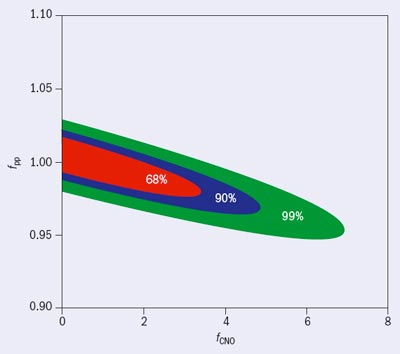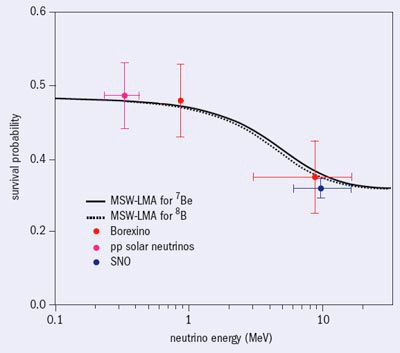Borexino homes in on neutrino oscillations
(CERN Courier)

Experiment at Gran Sasso provides key evidence for the solar-neutrino problem.

The mystery of the “missing” solar neutrinos arose in the 1970s when the pioneering experiment by Raymond Davis and colleagues in the Homestake Mine in South Dakota detected only one-third or so of the number of electron-neutrinos from the Sun that they expected. It was 30 years before this puzzle was solved, when the Sudbury Neutrino Observatory (SNO) confirmed the proposal that the neutrinos change type on their way from the centre of the Sun, reducing the number of electron-neutrinos arriving at the Earth. Such oscillations from one type to another can only occur if the neutrinos detected are mixtures of states with some difference in mass, in turn implying that neutrinos must have mass – a finding that lies beyond the Standard Model of particle physics.

Solar neutrinos have for the past 40 years been detected either by exploiting radiochemical techniques or by the detection of Cherenkov radiation. The Homestake detector exemplified the radiochemical method, with electron-neutrinos interacting with 37Cl to produce 37Ar, which was then extracted and detected through its radioactive decay. SNO, on the other hand, used heavy water to detect Cherenkov radiation from charged particles that were produced by neutrino interactions in the liquid. The results from all of the various experiments are best described by the theoretical description of neutrino oscillation developed by Stanislav Mikheyev, Alexei Smirnov and Lincoln Wolfenstein (MSW), and in particular the solution with a large mixing angle (LMA) between the mass states.

### Towards the MSW-LMA scenario

To explain the flux of electron-neutrinos relative to the total flux of solar neutrinos observed in SNO, as well as the results from Homestake and other experiments, the MSW-LMA mechanism requires two different regimes for neutrino oscillation: resonant, matter-enhanced oscillations in the dense core of the Sun for energies above 5 MeV (as in SNO); and vacuum-driven oscillations for low energies, below 2 MeV (as in the gallium radiochemical experiments, GALLEX, its successor the Gallium Neutrino Observatory and SAGE). Now, for the first time, the Borexino Experiment at the Gran Sasso National Laboratories has found experimental evidence for the transition between these two oscillation regimes by detecting in real time both low-energy (0.862 MeV) and high-energy (3–16 MeV) solar neutrinos, from 7Be and 8B, respectively. These nuclei are both formed in certain branches of the principal chain of reactions that converts hydrogen to helium at the Sun’s core – the so-called proton–proton (pp) chain, which starts with the pp process, p+p→d+e++ νe. While the 7Be neutrinos form 7% of the neutrinos that emanate from the Sun, the 8B neutrinos above 5 MeV correspond to only 0.006% of the total flux.

Borexino consists of an unsegmented liquid-scintillator detector with a target mass of 278 t of pseudocumene (C9H12) doped with 1.5 g/l of PPO (2,5 diphenyloxazole). The scintillator is contained inside a thin (125 μm) nylon vessel that is shielded against external background by a second nylon vessel and about 1 kt of buffer, which consists of pseudocumene mixed with 5 g/l of light quencher (dimethylphthalate). A total of 2212 8-inch photomultipliers mounted on a 13.7 m diameter stainless-steel sphere (SSS) detect the scintillator light. The SSS works as a containment vessel for both the scintillator and the buffer. It is installed inside a tank containing 2100 t of high-purity water.

### The 7Be measurement

One of the main research goals for Borexino is the detection of the solar neutrinos emanating from the electron-capture reactions of 7Be, which occurs in 15% of the conversions through the proton–proton chain. The 7Be neutrinos are monoenergetic (0.862 MeV, with a 90% branching ratio) and in Borexino they are detected via elastic scattering between neutrinos and electrons. The 7Be solar neutrinos offer a unique way to tag events: the kinematic Compton-like edge at 0.665 MeV. This is an important feature because solar-neutrino interactions cannot be disentangled from the residual beta-decay radioactivity arising from natural contaminants that are present in the scintillator. Figure 1 shows the expected solar-neutrino spectrum in Borexino, emphasizing the signal from the 7Be neutrinos.

The intrinsic radiopurity level of the scintillator is the main experimental challenge for such a detector. In Borexino, after five years of R&D, we developed purification methods that allowed us to achieve excellent purity, with intrinsic 238U and 232Th contamination levels of less than 1 in 1017. This level of radiopurity – a record in the field – allows us to study neutrino interactions in real time at, and below, 1 MeV. It also opens up new research windows such as:

• the possibility of detecting, in real time, neutrinos from the pep reaction and the CNO chain

• measuring low-energy 8B neutrinos through the reaction 13C(νe,e)13N

• searching for rare processes with very high sensitivity, such as probing the Pauli-exclusion principle at the level of >1030 y–1 by searching for non-Paulian transitions in 12C nuclei (Derbin 2008).

Borexino has been taking data since May 2007. After a few months a clear signal in the energy spectrum of events detected in the fiducial mass of about 80 t revealed the first detection of 7Be solar neutrinos (Borexino Collaboration, Arpesella et al. 2008). This observation allowed the first direct determination of the electron-neutrino survival probability, Pee, below 1 MeV. The MSW-LMA model predicts two regimes for Pee: namely, below 1 MeV, with Pee ˜0.6; and above 2 MeV, with Pee ˜0.3. Prior to Borexino only radiochemical experiments could probe the energy region below 1 MeV and they all measured an integrated solar-neutrino flux above a certain threshold – the threshold for the electron-neutrino capture interaction. The observation of 7Be neutrinos by Borexino provides a result of Pee=0.56±0.10 at 0.862 MeV, which is in good agreement with the MSW-LMA prediction (Borexino Collaboration, Alimonti et al. 2008).

This measurement casts light on another unresolved aspect of the physics of the solar core: the ratio of helium production via the pp chain and a cycle that involves carbon, nitrogen and oxygen (the CNO cycle). When taken all together, the integrated rates measured by Homestake and the gallium experiments are a function of the fluxes of solar neutrinos from pp, 7Be, the CNO cycle and the decay of 8B. Therefore, using the Borexino result on 7Be neutrinos, it is possible to study the correlation between the pp and CNO fluxes. Figure 2 shows contours at the 68%, 90% and 99% confidence levels for the combined estimate of the pp and CNO fluxes, normalized to the predictions of the Solar Standard Model (SMM). The 8B flux is fixed by the Cherenkov experiments (Super-Kamiokande and SNO).Fig. 2.  Contours at different confidence levels showing the correlation between the pp and CNO fluxes using the measurement by the Borexino experiment together with the luminosity constraint.

As figure 2 shows, the measurement of 7Be neutrinos is important for the study of a fundamental parameter, the flux of pp neutrinos, which are the most abundant solar neutrinos produced in the core of the Sun. The theory for beta-decay, with some extension, allows the calculation of the basic pp→d+e+νe cross-section, which at 1 MeV is around 10–47 cm2. Measuring such a small value is beyond the reach of current technology, so the cross-section for this important process – which drives the evolution of the Sun – can only be determined theoretically. A check of the flux predicted by the SMM for pp neutrinos is therefore important.

Figure 2 makes use of the luminosity constraint – a specific linear combination of solar-neutrino fluxes that corresponds to the measured photon-solar luminosity, assuming that nuclear-fusion reactions are responsible for generating energy inside the Sun. It leads to a value of fpp=1.04+0.13–0.19 with the luminosity constraint; without the constraint fpp=1.005+0.008–0.020. These are the best measurements of the pp solar-neutrino flux. The result on fCNO translates into a CNO contribution to the solar luminosity of <5.4% (90% CL); the current SMM predicts a contribution of order 1%.

Borexino has also recently performed a measurement of the 8B solar-neutrino flux above 3 MeV, which was possible because of the high radiopurity achieved. Prior to Borexino, 8B neutrinos were measured above 5 MeV using Cherenkov detectors. The results from these experiments agree well with Borexino’s measurement.Survival probability at Earth for electron solar neutrinos as a function of energy. The two Borexino measurements are shown together with the SNO result and the value predicted for pp neutrinos.

The measurement of the 8B flux allows a determination of the corresponding value of Pee at an effective energy (taking into account the spectrum of 8B neutrinos) of 8.6 MeV. So by detecting 8B neutrinos Borexino has measured Pee simultaneously at 0.862 MeV and at 8.6 MeV (figure 3). Disregarding systematic effects, which are the same for the measurement of Pee at low and high energies, the result determines a difference at about 2σ for Pee for 7Be and 8B neutrinos. The measured ratio of the survival probability for 7Be and 8B neutrinos is currently 1.60±0.33 (Borexino collaboration, Bellini et al. 2008). Using other solar-neutrino observations it is also possible to determine Pee for pp neutrinos, which figure 3 also shows. Combined, these results confirm for the first time the vacuum-matter transition predicted by the MSW-LMA scenario at today’s accuracy.

• Borexino at the Gran Sasso Laboratory is an international collaboration funded by INFN (Italy); NSF (US) for Princeton University, Virginia Tech, University of Massachusetts Amherst; BMBF and DFG (Germany) for MPI für Kernphysik Heidelberg, TU München; Rosnauka (Russia) for RRC Kurchatov Institute and JINR; MNiSW (Poland) for Institute of Physics Jagellonian University; and Laboratoire APC Paris.

Gianpaolo Bellini and Aldo Ianni, for the Borexino Collaboration.

Borexino homes in on neutrino oscillations